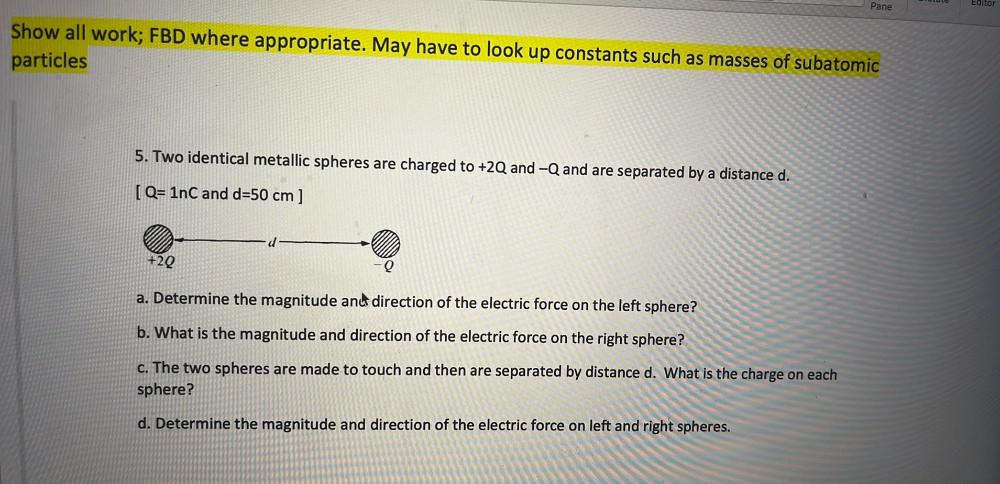Question:

# UUL Pane Editor Show all work; FBD where appropriate. May have to look up constants such as masses of subatomic particles 5. TwoUUL Pane Editor Show all work; FBD where appropriate. May have to look up constants such as masses of subatomic particles 5. Two identical metallic spheres are charged to +2Q and -Q and are separated by a distance d. [Q= 1nC and d=50 cm ] +29 Q a. Determine the magnitude and direction of the electric force on the left sphere? b. What is the magnitude and direction of the electric force on the right sphere? C. The two spheres are made to touch and then are separated by distance d. What is the charge on each sphere? d. Determine the magnitude and direction of the electric force on left and right spheres.